# Convert Date to Number (or Number to Date) – Excel & Google Sheets

This tutorial will demonstrate how to convert a date to a serial number corresponding to that date (or vis-a-versa) in Excel and Google Sheets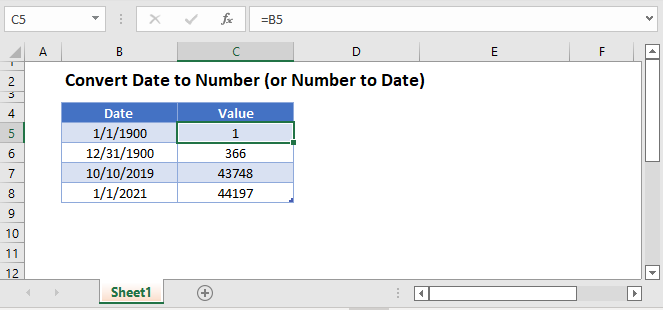## Date to Number

To convert a date to a serial number, all you need to do is change the formatting to General: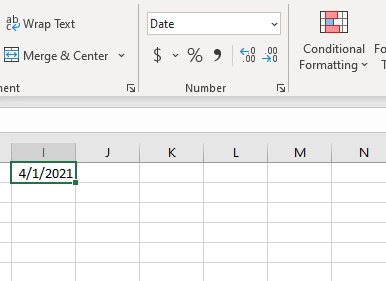However, this won’t work if the date is stored as text.

### Date Stored as Text to Number

If the date is stored as text, you can use DATEVALUE convert it to an Excel date:

``=DATEVALUE(B3)``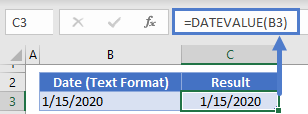Then you can change the formatting to general to see the serial number.

## Number to Date

To convert a serial number to a date, all you need to do is the change the formatting from General (or Number) to a date format (ex. Short Date):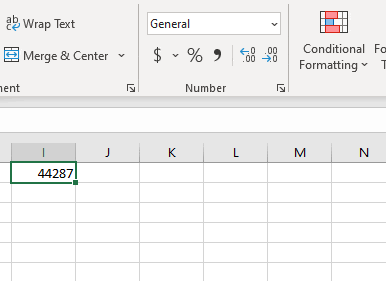## Convert Date to Number in Google Sheets

All of the above examples work exactly the same in Google Sheets as in Excel.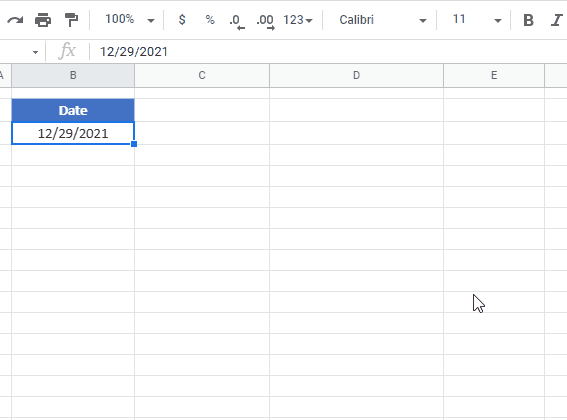### Excel Practice Worksheet

Practice Excel functions and formulas with our 100% free practice worksheets!